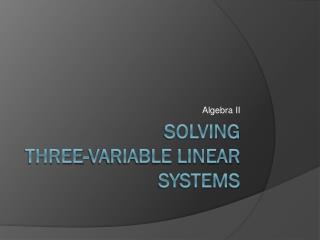DownloadDownload PresentationSolving three-variable linear systems

# Solving three-variable linear systems

Download Presentation## Solving three-variable linear systems

- - - - - - - - - - - - - - - - - - - - - - - - - - - E N D - - - - - - - - - - - - - - - - - - - - - - - - - - -
##### Presentation Transcript

1. Algebra II Solving three-variable linear systems

2. Solving 3-variable linear systems • Step 1: Create a 2 x 2 system • Pick two of the three equations and eliminate one variable. Make note of the two variables remaining. • Pick the remaining equation and one of the others to create a 2nd equation in the same two variables as the one in step 1a. • Step 2: Use the two 2 x 2 systems created in Step 1 to eliminate another variable.

3. Solving 3-variable linear systems • Step 3: Plug in the value found in step 2 into one of the 2 x 2 equations and solve for the other variable. • Step 4: Plug the two values you’ve already found back into one of the original equations to find the value of the remaining variable. • Step 5: Write your solution as an ordered triple (x,y,z).